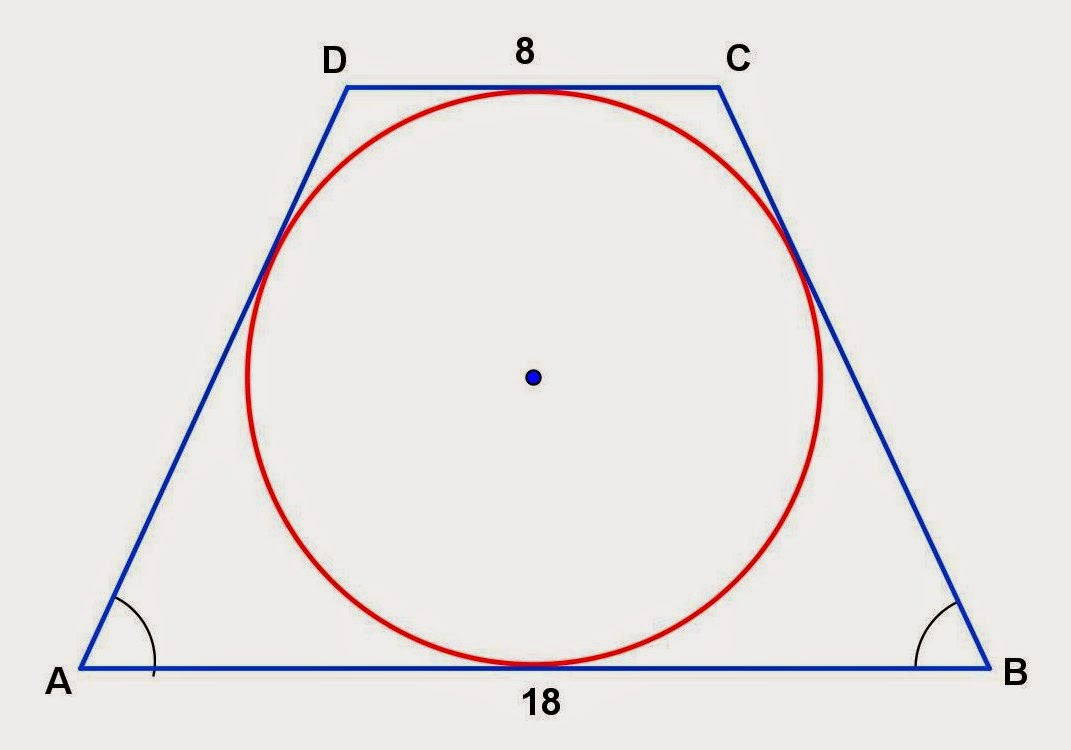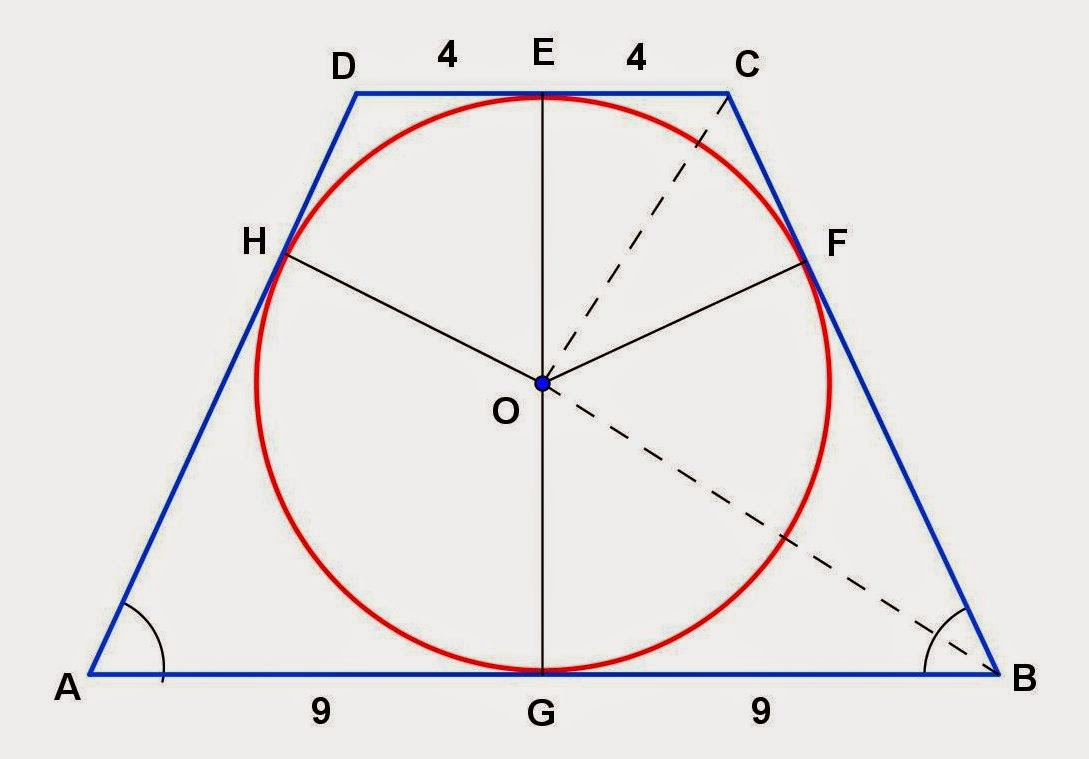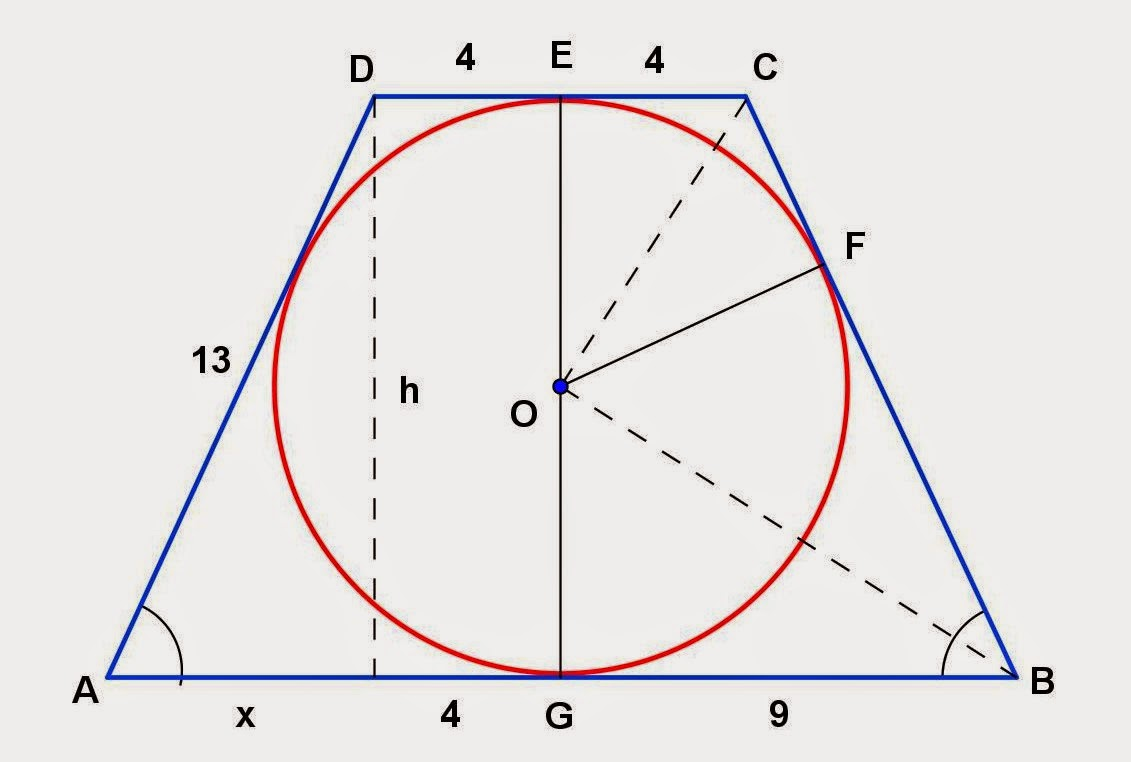## Tuesday, December 23, 2014

### Circle Inscribed in a Trapezoid Problems

Category: Plane Geometry

"Published in Newark, California, USA"

Find the exact area of the given trapezoid in the figure:Photo by Math Principles in Everyday Life

Solution:

Consider the given figure abovePhoto by Math Principles in Everyday Life

Since the given figure is an isosceles trapezoid, then it follows that ∠A ≅ ∠B, ∠C ≅ ∠D, and AD ≅ BC. If a circle is inscribed in an isosceles trapezoid, then its radius is tangent to the sides of an isosceles trapezoid. Let's analyze and label further the given figure as followsPhoto by Math Principles in Everyday Life

Line segment EG that passes the center of a circle bisects the two bases of an isosceles trapezoid. Line segment OB bisects ∠B and line segment OC bisects ∠C.

Consider rt. ∆OGB and rt. ∆OFG. If OG ≅ OF and OB ≅ OB, then it follows that BG ≅ BF. In this case, BF = 9.

Consider rt. ∆OEC and rt. ∆OFC. If OE ≅ OF and OC ≅ OC, then it follows that EC ≅ CF. In this case, CF = 4.

Hence, the length of CB = CF + FB = 9 + 4 = 13.Photo by Math Principles in Everyday Life

The value of x is

By Pythagorean Theorem, the altitude of an isosceles trapezoid is

Therefore, the area of an isosceles trapezoid is